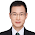## Thursday, September 27, 2012

### Sampling Rate is Not Only about Pixels: How to compare the sampling rate between your camera and "single-pixel" camera

At the beginning, I'd like to make clear two terms: "Nyquist frequency" and "Nyquist rate".
Some of you may take them as the same thing, and even some textbooks did so. However, although they are quite similar, they are actually different.
Reference: http://en.wikipedia.org/wiki/Nyquist_frequency
Nyquist Frequency: A property of system. For a given system, sampling rate $f_s$ is fixed. The Nyquist frequency of the system is the allowed highest frequency of a signal that could be sampled without aliasing, which is $f_s / 2$.
Nyquist Rate: A property of signal. For a given band-limited or band-pass signal, bandwidth B is fixed. The Nyquist rate of the signal is the necessary lowest sampling frequency of a system that could sample the signal without aliasing, which is $2B$.

Then for an (imaging system, image signals), you can interpret the sampling rate is the number of pixels, the highest frequency of the image is the resolution of the image.
Then the Nyquist says here, for a imaging system, the number of pixels (sampling rate) is fixed, the highest allowed resolution of the pixel is determined by/proportional to the number of pixels. For different kinds of measure of resolution, the proportion factor differs.
Meanwhile, for an image signal, whose resolution (band-width) is fixed, the required pixels is then determined by/proportional to the resolution.

So the number of pixels is actually the number of samples a system takes for a given image.
Then does it mean "single-pixel" means the number of samples is 1? The answer is definitely no.
So how to interpret the "single"-pixel?

It's a little tricky, but still can be understood. The only thing you need to do is adding a time-axis.
The traditional imaging system in your cameras actually taking samples at one time, each sample is a pixel. So the total number of samples is (number of pixels in a unit of time) x (unit of time). Since the (unit of time) is 1 here, we usually ignore it.
For the "signal-pixel" camera, the number of samples is (number of pixels in a unit of time) x (unit of time). Here (the number of pixels in a unit of time) is 1, whereas the (unit of time) is not 1, which determines the number of samples.
Image sensors are silicon chips that capture and read light. So in traditional imaging system, we need thousands of image sensors, whereas in single pixel camera, we only use one image sensor to capture and read light.
That's the trade-off, number of pixels (image sensors) and unit of time (exposure time).

Therefore, improved interpretation of Nyquist sampling here is to consider the sampling rate being (number of pixels in a unit of time) x (unit of time), i.e., the product of space sampling and time sampling.

#### 1 comment:

1.